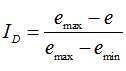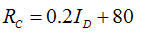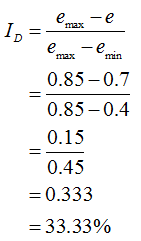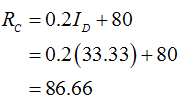# Determine the density index of a soil having a natural void ratio 0.7. The void ratio of the soil in the loosest and most dense state is 0.85 and 0.4 respectively. Also, calculate the relative compaction.

Question
1 views

Determine the density index of a soil having a natural void ratio 0.7. The void ratio of the soil in the loosest and most dense state is 0.85 and 0.4 respectively. Also, calculate the relative compaction.

check_circle

star
star
star
star
star
1 Rating
Step 1

Given:

Void ratio of soil, e = 0.7

Void ratio of soil in loosest state, emax = 0.85

Void ratio of soil in most dense state, emin = 0.4

Step 2

The formula for relative density is given by,The formula for relative compaction is given by,Step 3

The relative density is calculated as,The relative compaction is calculated as,...

### Want to see the full answer?

See Solution

#### Want to see this answer and more?

Solutions are written by subject experts who are available 24/7. Questions are typically answered within 1 hour.*

See Solution
*Response times may vary by subject and question.
Tagged in